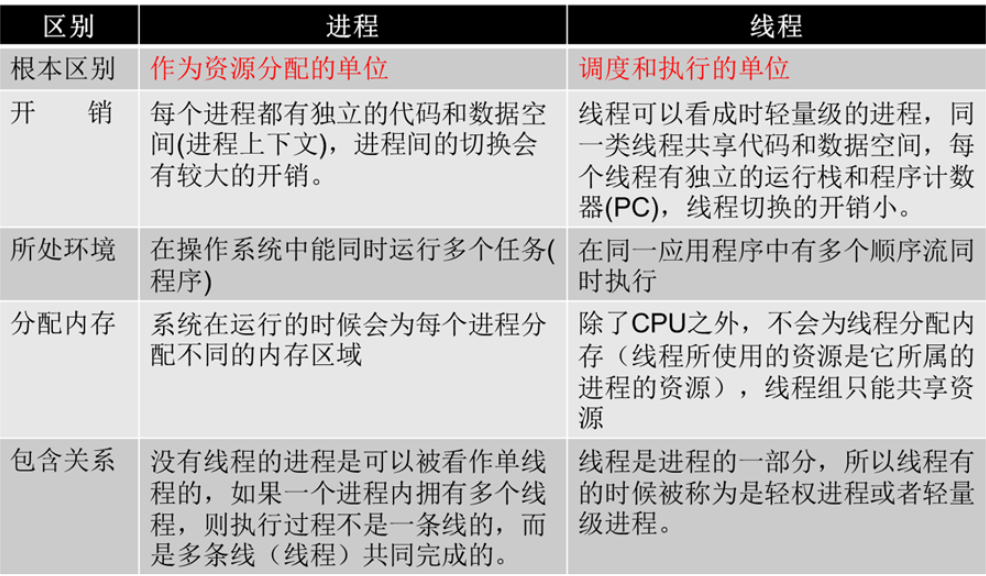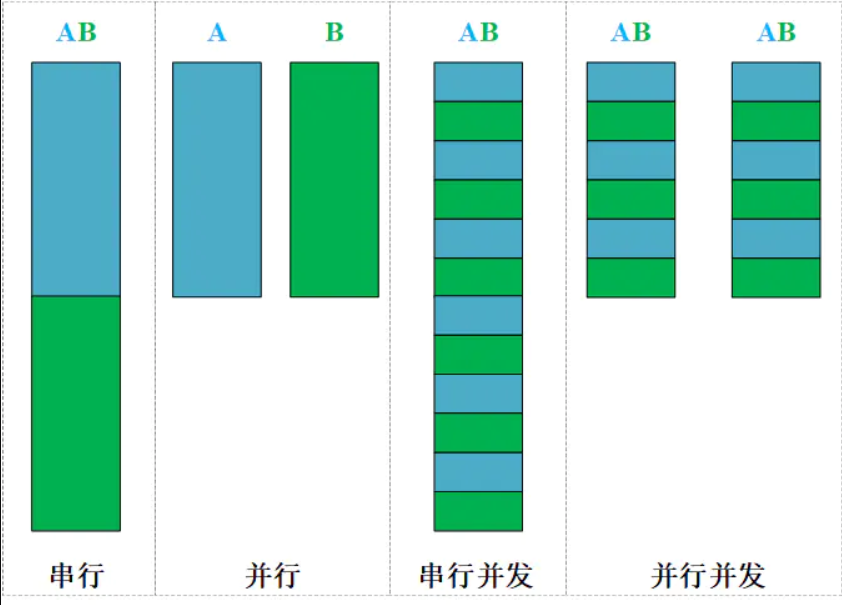# 多任务处理方式之二：多线程

### 线程的理解

• 1、操作系统能够进行运算调度的最小单位，即程序执行的最小单位

• 2、进程负责程序所必须的资源分配（文本区域、数据区域、堆栈区域）一个进程中也经常需要同时做多件事，即要同时运行多个‘子任务’，这些子任务即线程

线程是每一个进程中的单一顺序控制流 ,其包含在进程中，是进程的实际运作单位（进程是线程的容器）

⼀个程序中⾄少要有⼀个进程，⼀个进程中⾄少要有⼀个线程

线程不能够独⽴执⾏，必须依存在进程中

• 3、线程基本不占用系统资源，其只拥有在运行过程中必不可少的资源（如程序计数器、一组寄存器和栈）

• 4、同一个进程中的所有线程都共享此进程所拥有的全部资源

一个进程中的线程共享相同的内存单元/内存地址空间——>可以访问相同的变量和对象，而且它们从同一堆中分配对象——>通信、数据交换、同步操作

• 5、线程之间的通信主要通过共享所属进程的资源

线程间的通信是在同一地址空间上进行的，所以不需要额外的通信机制，这就使得通信更简便而且信息传递的速度也更快

• 6、线程的上下文切换很快，资源开销较少，但是相对于进程而言，不够安全，在多个线程共同操作进程的某一资源时，可能会丢失数据

• 7、线程和进程之间的区别### 线程的五种状态

1. 新状态：线程对象已经创建，还未调用 `start()` 方法。
1. 可运行状态：当线程有资格运行，但调度程序还没有把它选定为运行线程时线程所处的状态。当 `start()`方法调用时，线程首先进入可运行状态。在线程运行之后或者从阻塞、等待或睡眠状态回来后，也返回到可运行状态。
1. 运行状态：线程调度程序从可运行池中选择一个线程作为当前线程时线程所处的状态。这也是线程进入运行状态的唯一方式。
1. 等待/阻塞/睡眠状态：这是线程有资格运行时它所处的状态。实际上这个三状态组合为一种，其共同点是：线程仍旧是活的（可运行的），但是当前没有条件运行。但是如果某件事件出现，他可能返回到可运行状态。
1. 死亡态：当线程的`run()`方法完成时就认为它死去。这个线程对象也许是活的，但是，它已经不是一个单独执行的线程。线程一旦死亡，就不能复生。如果在一个死去的线程上调用 `start()`方法，会抛出 `java.lang.IllegalThreadStateException` 异常。

### GIL全局解释器锁

Python中的多线程可以并发，但不能并行（同一个进程下的多个线程不能被多个cpu同时执行），缘由就是GIL全局解释器锁，导致同一时间内只有一个线程在执行

python 文件的执行流程为：

• 操作系统先将python解释器和需要执行的py文件由硬盘加载到内存中，开辟一个进程空间
• 此进程即使得python解释器首先将py文件中的代码指令通过编译器编译成字节码
• 编译完成的c的字节码通过虚拟机转换为机器码由cpu执行

### 线程创建

`from threading import Thread`

``````from threading import Thread
import os

def func(num):
print('当前线程{},所归属的进程id号{}'.format(os.getpid(), num))

for i in range(10):
# 异步创建10个子线程
t.start()

# 主线程执行任务
print(os.getpid())
``````

``````from threading import Thread
import time

def __init__(self, name):
# 手动调用父类的构造方法
super().__init__()
self.name = name

def run(self):
time.sleep(1)
print("当前线程正在执行runing ... ", self.name)

if __name__ == "__main__":
t.start()
print("主线程执行结束 ... ")
``````

• t.is_alive() 检测线程是否仍然存在

• t.setName() 设置线程名字

• t.getName() 获取线程名字

``````from threading import Thread
import time

def func():
time.sleep(1)

if __name__ == "__main__":

t.start()
print(t , type(t))

print(t.is_alive())  # False

print(t.getName())

t.setName("xboyww")
print(t.getName())

``````
• enumerate() 返回目前正在运行的线程列表
• activeCount() 返回目前正在运行的线程数量
``````from threading import Thread
import time

def func():

if __name__ == "__main__":

# 2.enumerate()        返回目前正在运行的线程列表

def func():
time.sleep(0.5)

if __name__ == "__main__":
for i in range(10):
lst = enumerate()
# 子线程10 + 主线程1个 = 11
print(lst ,len(lst))

# 3.activeCount()      返回目前正在运行的线程数量
print(activeCount())
``````

``````from concurrent.futures import ThreadPoolExecutor

def func(i):
time.sleep(3)

if __name__ == "__main__":
lst = []
setvar = set()
# (1) 创建线程池对象
"""限制线程池最多创建os.cpu_count() * 5 = 线程数,所有任务全由这几个线程完成,不会额外创建线程"""

# (2) 异步提交任务
for i in range(100):
res = tp.submit(func, i)
lst.append(res)

# (3) 获取返回值
for i in lst:

# (4) 等待所有子线程执行结束
tp.shutdown()

print(len(setvar), setvar)
print("主线程执行结束 ... ")

``````

### 守护线程

`线程对象.setDaemon(True)`

``````from threading import Thread
import time

def func1():
while True:
time.sleep(0.5)
print("我是func1")

def func2():
print("我是func2 start ... ")
time.sleep(3)
print("我是func2 end ... ")

# 在start调用之前,设置守护线程
t1.setDaemon(True)

t1.start()
t2.start()

print("主线程执行结束 ... ")
``````

### 同步 & 异步

#### 同步

• 场景1：是指完成事务的逻辑，先执行第一个事务，如果阻塞了，会一直等待，直到这个事务完成，再执行第二个事务，协同步调，按预定的先后次序进行运行

.NETCore中实现ObjectId反解

• 场景2：一个任务的完成需要依赖另外一个任务时，只有等待被依赖的任务完成后，依赖的任务才能算完成，这是一种可靠的任务序列

#### 异步

• 处理调用这个事务之后，不会等待这个事务的处理结果，直接处理第二个事务去了，通过状态、回调来通知调用者处理结果

• 对于I/O相关的程序来说，异步编程可以大幅度的提高系统的吞吐量，因为在某个I/O操作的读写过程中，系统可以先去处理其它的操作（通常是其它的I/O操作）

• 不确定执行顺序

### 串行 & 并行 & 并发

#### 串行

A和B两个任务运行在一个CPU线程上，在A任务执行完之前不可以执行B。即，在整个程序的运行过程中，仅存在一个运行上下文，即一个调用栈一个堆。程序会按顺序执行每个指令

#### 图示### 线程同步

``````import threading
import time

def work1(nums):
nums.append(44)
print('-----in work1-----', nums)

def work2(nums):
time.sleep(1)
# 延时一会保证另一线程执行
print('-----in work2-----', nums)

g_nums = [11, 22, 33]
t1.start()
t2.start()

``````

``````import threading

num = 0

def test1():
global num

# 调用Lock对象的acquire()方法获得锁时，这把锁进入“locked”状态
# 如果此时另一个线程2试图获得这个锁，该线程2就会变为同步阻塞状态
if mutex.acquire():
for i in range(1000):
num += 1

# 调用Lock对象的release()方法释放锁之后，该锁进入“unlocked”状态。
mutex.release()

def test2():
global num

# 线程调度程序继续从处于同步阻塞状态的线程中选择一个来获得锁，并使得该线程进入运行（running）状态
if mutex.acquire():
for i in range(1000):
num += 1
mutex.release()

p1.start()
p2.start()
print(num)
``````
##### 死锁（只上锁，不解锁）

``````import threading
import time

def run(self):
# 线程1被 A 锁——>锁定
if mutexA.acquire():
print(self.name + '---do1---up---')
time.sleep(1)
if mutexB.acquire():
print(self.name + '---do1---down---')
mutexB.release()

# 线程1被 A 锁释放的前提是：线程1 抢到 B 锁
mutexA.release()

def run(self):
time.sleep(1)
# 线程2被 B 锁——>锁定
if mutexB.acquire():
print(self.name + '---do2---up---')
if mutexA.acquire():
print(self.name + '---do2---down---')
mutexA.release()

# 线程2被 B 锁释放的前提是：线程2 抢到 A 锁
mutexB.release()

if __name__ == '__main__':
t1.start()
t2.start()

# 程序卡死

# 线程1不释放A锁
# 线程2不释放B锁

``````

``````import threading
import time

def run(self):
if mutexA.acquire():
print(self.name + '---do1---up---')
time.sleep(1)
if mutexB.acquire():
print(self.name + '---do1---down---')
mutexB.release()
mutexA.release()

def run(self):
time.sleep(1)
if mutexB.acquire():
print(self.name + '---do2---up---')
if mutexA.acquire():
print(self.name + '---do2---down---')
mutexA.release()

mutexB.release()

if __name__ == '__main__':
t1.start()
t2.start()

``````

``````import time

def foo(se):
se.acquire()
time.sleep(2)
print("ok")
se.release()

if __name__ == "__main__":
# 设置同一时间内可以有5个线程并发

for i in range(20):
t1.start()  # 此时可以控制同时进入的线程数
``````

#### 线程队列（queue模块）

##### queue.Queue：FIFO（先⼊先出) 队列 Queue
``````# 基本使用
from queue import Queue

# put 存
# get 取
# put_nowait 存,超出了队列长度,报错
# get_nowait 取,没数据取不出来,报错

# linux windows 线程中put_nowait,get_nowait都支持

"""先进先出,后进后出"""
# maxsize为一个整数,表示队列的最大条目数，可用来限制内存的使用。
# 一旦队列满，插入将被阻塞直到队列中存在空闲空间。如果maxsize小于等于0，队列大小为无限。maxsize默认为0

q = Queue(maxsize=0)
q.put(1)
q.put(2)
print(q.get())
print(q.get())
# 取不出来,阻塞
# print(q.get())
print(q.get_nowait())

q2 = Queue(3)
q2.put(11)
q2.put(22)
q2.put(33)
# 放不进去了,阻塞
# q2.put(44)
q2.put_nowait(44)
``````
``````import threading
import time
from queue import Queue

def run(self):
global queue
count = 0
while True:
if queue.qsize() < 1000:
for i in range(100):
count = count + 1
msg = '生成产品' + str(count)
queue.put(msg)  # 队列中添加新产品
print(msg)
time.sleep(1)

def run(self):
global queue
while True:
if queue.qsize() > 100:
for i in range(3):
msg = self.name + '消费了' + queue.get()
print(msg)
time.sleep(1)

if __name__ == "__main__":
queue = Queue()
# 创建一个队列。线程中能用，进程中不能使用
for i in range(500):  # 创建500个产品放到队列里
queue.put('初始产品' + str(i))  # 字符串放进队列
for i in range(2):  # 创建了两个线程
p = Pro()
p.start()
for i in range(5):  # 5个线程
c = Con()
c.start()

``````
##### queue.LifoQueue：LIFO（后⼊先出） 栈 LifoQueue
``````# LifoQueue 先进后出,后进先出(按照栈的特点设计)

from queue import LifoQueue

lq = LifoQueue(3)
lq.put(11)
lq.put(22)
lq.put(33)
# print(lq.put_nowait(444))

print(lq.get())
print(lq.get())
print(lq.get())
``````
##### queue.PriorityQueue：（优先级队列） PriorityQueue
``````# PriorityQueue 按照优先级顺序排序 (默认从小到大排序)

from queue import PriorityQueue

# 如果都是数字,默认从小到大排序
pq = PriorityQueue()
pq.put(13)
pq.put(3)
pq.put(20)
print(pq.get())
print(pq.get())
print(pq.get())

# 如果都是字符串
"""如果是字符串,按照ascii编码排序"""
pq1 = PriorityQueue()
pq1.put("chinese")
pq1.put("america")
pq1.put("latinos")
pq1.put("blackman")

print(pq1.get())
print(pq1.get())
print(pq1.get())
print(pq1.get())

# 要么全是数字,要么全是字符串,不能混合 error
"""
pq2 = PriorityQueue()
pq2.put(13)
pq2.put("aaa")
pq2.put("拟稿")
"""

pq3 = PriorityQueue()
# 默认按照元组中的第一个元素排序
pq3.put( (20,"wangwen") )
pq3.put( (18,"wangzhen") )
pq3.put( (30,"weiyilin") )
pq3.put( (40,"xiechen") )

print(pq3.get())
print(pq3.get())
print(pq3.get())
print(pq3.get())
``````

#### 生产消费者模式

• 进程（线程）之间如果直接通信，可能会出现两个问题

• 耦合性太强
• 速率有可能不匹配

解决方式,找一个缓冲区来中转数据即生产者——消费者模式

### 线程异步

#### 通过回调函数可以实现多线程异步执行

``````from concurrent.futures import ProcessPoolExecutor, ThreadPoolExecutor
import os, time

def func1(i):
print("Process start ... ", os.getpid())
time.sleep(0.5)
print("Process end ... ", i)
return "*" * i

def func2(i):
time.sleep(0.5)
return "*" * i

def call_back1(obj):
print("<==回调函数callback进程号:===>", os.getpid())
print(obj.result())

def call_back2(obj):
print(obj.result())

# (1) 进程池的回调函数: 由主进程执行调用完成

if __name__ == "__main__":
p = ProcessPoolExecutor(5)
for i in range(1, 11):
res = p.submit(func1, i)
'''
'''
p.shutdown()
print("主进程执行结束 ... ", os.getpid())

# (2) 线程池的回调函数: 由当前子线程执行调用完成
if __name__ == "__main__":
for i in range(1, 11):
res = tp.submit(func2, i)
'''
'''
tp.shutdown()
``````
``````from multiprocessing import Pool
import random
import time

for i in range(1, 4):
print(f"{f}下载文件{i}")
time.sleep(random.randint(1, 3))
return "下载完成"

def alterUser(msg):
print(msg)

if __name__ == "__main__":
p = Pool(3)
# 当func执行完毕后，return的东西会给到回调函数callback